Algebra Commons™

1,047 Full-Text Articles 1,224 Authors 224,226 Downloads118 Institutions

All Articles in Algebra

1,047 full-text articles. Page 6 of 39.

Application Of Jordan Algebra For Testing Hypotheses About Structure Of Mean Vector In Model With Block Compound Symmetric Covariance Structure, 2018Faculty of Mathematics, Computer Science and Econometrics, University of Zielona Góra,

Application Of Jordan Algebra For Testing Hypotheses About Structure Of Mean Vector In Model With Block Compound Symmetric Covariance Structure, Roman Zmyślony, Ivan Zezula, Arkadiusz Kozioł

Electronic Journal of Linear Algebra

In this article authors derive test for structure of mean vector in model with block compound symmetric covariance structure for two-level multivariate observations. One possible structure is so called structured mean vector when its components remain constant over sites or over time points, so that mean vector is of the form $\boldsymbol{1}_{u}\otimes\boldsymbol{\mu}$ with $\boldsymbol{\mu}=(\mu_1,\mu_2,\ldots,\mu_m)'\in\mathbb{R}^m$. This hypothesis is tested against alternative of unstructured mean vector, which can change over sites or over time points.

Inertia Sets Allowed By Matrix Patterns, 2018Saint Olaf College

Inertia Sets Allowed By Matrix Patterns, Adam H. Berliner, Dale D. Olesky, Pauline Van Den Driessche

Electronic Journal of Linear Algebra

Motivated by the possible onset of instability in dynamical systems associated with a zero eigenvalue, sets of inertias $\sn_n$ and $\SN{n}$ for sign and zero-nonzero patterns, respectively, are introduced. For an $n\times n$ sign pattern $\mc{A}$ that allows inertia $(0,n-1,1)$, a sufficient condition is given for $\mc{A}$ and every superpattern of $\mc{A}$ to allow $\sn_n$, and a family of such irreducible sign patterns for all $n\geq 3$ is specified. All zero-nonzero patterns (up to equivalence) that allow $\SN{3}$ and $\SN{4}$ are determined, and are described by their associated digraphs.

Some Graphs Determined By Their Distance Spectrum, 2018Department of mathematics & statistics, McGill University, Montreal

Some Graphs Determined By Their Distance Spectrum, Stephen Drury, Huiqiu Lin

Electronic Journal of Linear Algebra

Let $G$ be a connected graph with order $n$. Let $\lambda_1(D(G))\geq \cdots\geq \lambda_n(D(G))$ be the distance spectrum of $G$. In this paper, it is shown that the complements of $P_n$ and $C_n$ are determined by their $D$-spectrum. Moreover, it is shown that the cycle $C_n$ ($n$ odd) is also determined by its $D$-spectrum.

A Tensor's Torsion, 2018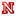University of Nebraska-Lincoln

A Tensor's Torsion, Neil Steinburg

Dissertations, Theses, and Student Research Papers in Mathematics

While tensor products are quite prolific in commutative algebra, even some of their most basic properties remain relatively unknown. We explore one of these properties, namely a tensor's torsion. In particular, given any finitely generated modules, M and N over a ring R, the tensor product $M\otimes_R N$ almost always has nonzero torsion unless one of the modules M or N is free. Specifically, we look at which rings guarantee nonzero torsion in tensor products of non-free modules over the ring. We conclude that a specific subclass of one-dimensional Gorenstein rings will have this property.

Adviser: Roger Wiegand ...

Partially-Ordered Multi-Type Algebras, Display Calculi And The Category Of Weakening Relations, 2018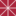Chapman University

Partially-Ordered Multi-Type Algebras, Display Calculi And The Category Of Weakening Relations, Peter Jipsen, Fei Liang, M. Andrew Moshier, Apostolos Tzimoulis

Mathematics, Physics, and Computer Science Faculty Articles and Research

"We define partially-ordered multi-type algebras and use them as algebraic semantics for multi-type display calculi that have recently been developed for several logics, including dynamic epistemic logic , linear logic, lattice logic , bilattice logic  and semi-De Morgan logic ."

Factorization In Integral Domains., 2018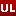University of Louisville

Factorization In Integral Domains., Ryan H. Gipson

Electronic Theses and Dissertations

We investigate the atomicity and the AP property of the semigroup rings F[X; M], where F is a field, X is a variable and M is a submonoid of the additive monoid of nonnegative rational numbers. In this endeavor, we introduce the following notions: essential generators of M and elements of height (0, 0, 0, . . .) within a cancellative torsion-free monoid Γ. By considering the latter, we are able to determine the irreducibility of certain binomials of the form Xπ − 1, where π is of height (0, 0, 0, . . .), in the monoid domain. Finally, we will consider relations between the ...

Developments In Multivariate Post Quantum Cryptography., 2018University of Louisville

Developments In Multivariate Post Quantum Cryptography., Jeremy Robert Vates

Electronic Theses and Dissertations

Ever since Shor's algorithm was introduced in 1994, cryptographers have been working to develop cryptosystems that can resist known quantum computer attacks. This push for quantum attack resistant schemes is known as post quantum cryptography. Specifically, my contributions to post quantum cryptography has been to the family of schemes known as Multivariate Public Key Cryptography (MPKC), which is a very attractive candidate for digital signature standardization in the post quantum collective for a wide variety of applications. In this document I will be providing all necessary background to fully understand MPKC and post quantum cryptography as a whole. Then ...

On N/P-Asymptotic Distribution Of Vector Of Weighted Traces Of Powers Of Wishart Matrices, 2018Linnaeus University, Växjö, Sweden

On N/P-Asymptotic Distribution Of Vector Of Weighted Traces Of Powers Of Wishart Matrices, Jolanta Maria Pielaszkiewicz, Dietrich Von Rosen, Martin Singull

Electronic Journal of Linear Algebra

The joint distribution of standardized traces of $\frac{1}{n}XX'$ and of $\Big(\frac{1}{n}XX'\Big)^2$, where the matrix $X:p\times n$ follows a matrix normal distribution is proved asymptotically to be multivariate normal under condition $\frac{{n}}{p}\overset{n,p\rightarrow\infty}{\rightarrow}c>0$. Proof relies on calculations of asymptotic moments and cumulants obtained using a recursive formula derived in Pielaszkiewicz et al. (2015). The covariance matrix of the underlying vector is explicitely given as a function of $n$ and $p$.

2018University of Central Florida

A Note On The Matrix Arithmetic-Geometric Mean Inequality, Teng Zhang

Electronic Journal of Linear Algebra

This note proves the following inequality: If $n=3k$ for some positive integer $k$, then for any $n$ positive definite matrices $\bA_1,\bA_2,\dots,\bA_n$, the following inequality holds: \begin{equation*}\label{eq:main} \frac{1}{n^3} \, \Big\|\sum_{j_1,j_2,j_3=1}^{n}\bA_{j_1}\bA_{j_2}\bA_{j_3}\Big\| \,\geq\, \frac{(n-3)!}{n!} \, \Big\|\sum_{\substack{j_1,j_2,j_3=1,\\\text{$j_1$, $j_2$, $j_3$ all distinct}}}^{n}\bA_{j_1}\bA_{j_2}\bA_{j_3}\Big\|, \end{equation*} where $\|\cdot\|$ represents the operator norm. This inequality is a special case of a recent conjecture proposed by Recht and R ...

Local Higher Category Theory, 2018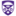The University of Western Ontario

Local Higher Category Theory, Nicholas Meadows

Electronic Thesis and Dissertation Repository

The purpose of this thesis is to give presheaf-theoretic versions of three of the main extant models of higher category theory: the Joyal, Rezk and Bergner model structures. The construction of these model structures takes up Chapters 2, 3 and 4 of the thesis, respectively. In each of the model structures, the weak equivalences are local or ‘stalkwise’ weak equivalences. In addition, it is shown that certain Quillen equivalences between the aforementioned models of higher category theory extend to Quillen equivalences between the various models of local higher category theory.

Throughout, a number of features of local higher category theory ...

Webwork Problems For Linear Algebra, 2018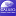University of North Georgia

Webwork Problems For Linear Algebra, Hashim Saber, Beata Hebda

Mathematics Ancillary Materials

This set of problems for Linear Algebra in the open-source WeBWorK mathematics platform was created under a Round Eleven Mini-Grant for Ancillary Materials Creation. The problems were created for an implementation of the CC-BY Lyrix open textbook A First Course in Linear Algebra. Also included as an additional file are the selected and modified Lyryx Class Notes for the textbook.

Topics covered include:

• Linear Independence
• Linear Transformations
• Matrix of a Transformation
• Isomorphisms
• Eigenvalues and Eigenvectors
• Diagonalization
• Orthogonality

Determining The Determinant, 2018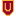Xavier University

Determining The Determinant, Danny Otero

Linear Algebra

No abstract provided.

Dimers On Cylinders Over Dynkin Diagrams And Cluster Algebras, 2018Louisiana State University and Agricultural and Mechanical College

Dimers On Cylinders Over Dynkin Diagrams And Cluster Algebras, Maitreyee Chandramohan Kulkarni

LSU Doctoral Dissertations

This dissertation describes a general setting for dimer models on cylinders over Dynkin diagrams which in type A reduces to the well-studied case of dimer models on a disc. We prove that all Berenstein--Fomin--Zelevinsky quivers for Schubert cells in a symmetric Kac--Moody algebra give rise to dimer models on the cylinder over the corresponding Dynkin diagram. We also give an independent proof of a result of Buan, Iyama, Reiten and Smith that the corresponding superpotentials are rigid using the dimer model structure of the quivers.

Rank Function And Outer Inverses, 2018Manipal University, Manipal

Rank Function And Outer Inverses, Manjunatha Prasad Karantha, K. Nayan Bhat, Nupur Nandini Mishra

Electronic Journal of Linear Algebra

For the class of matrices over a field, the notion of rank of a matrix' as defined by the dimension of subspace generated by columns of that matrix' is folklore and cannot be generalized to the class of matrices over an arbitrary commutative ring. The determinantal rank' defined by the size of largest submatrix having nonzero determinant, which is same as the column rank of given matrix when the commutative ring under consideration is a field, was considered to be the best alternative for the rank' in the class of matrices over a commutative ring. Even this determinantal rank and ...

Correlation Matrices With The Perron Frobenius Property, 2018Wilfrid Laurier University

Correlation Matrices With The Perron Frobenius Property, Phelim P. Boyle, Thierno B. N'Diaye

Electronic Journal of Linear Algebra

This paper investigates conditions under which correlation matrices have a strictly positive dominant eigenvector. The sufficient conditions, from the Perron-Frobenius theorem, are that all the matrix entries are positive. The conditions for a correlation matrix with some negative entries to have a strictly positive dominant eigenvector are examined. The special structure of correlation matrices permits obtaining of detailed analytical results for low dimensional matrices. Some specific results for the $n$-by-$n$ case are also derived. This problem was motivated by an application in portfolio theory.

Simple Groups, Progenitors, And Related Topics, 2018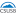California State University - San Bernardino

Simple Groups, Progenitors, And Related Topics, Angelica Baccari

Electronic Theses, Projects, and Dissertations

The foundation of the work of this thesis is based around the involutory progenitor and the finite homomorphic images found therein. This process is developed by Robert T. Curtis and he defines it as 2^{*n} :N {pi w | pi in N, w} where 2^{*n} denotes a free product of n copies of the cyclic group of order 2 generated by involutions. We repeat this process with different control groups and a different array of possible relations to discover interesting groups, such as sporadic, linear, or unitary groups, to name a few. Predominantly this work was produced from transitive ...

Galois Theory And The Quintic Equation, 2018Union College

Galois Theory And The Quintic Equation, Yunye Jiang

Honors Theses

Most students know the quadratic formula for the solution of the general quadratic polynomial in terms of its coefficients. There are also similar formulas for solutions of the general cubic and quartic polynomials. In these three cases, the roots can be expressed in terms of the coefficients using only basic algebra and radicals. We then say that the general quadratic, cubic, and quartic polynomials are solvable by radicals. The question then becomes: Is the general quintic polynomial solvable by radicals? Abel was the first to prove that it is not. In turn, Galois provided a general method of determining when ...

Symmetric Presentations, Representations, And Related Topics, 2018California State University - San Bernardino

Symmetric Presentations, Representations, And Related Topics, Adam Manriquez

Electronic Theses, Projects, and Dissertations

The purpose of this thesis is to develop original symmetric presentations of finite non-abelian simple groups, particularly the sporadic simple groups. We have found original symmetric presentations for the Janko group J1, the Mathieu group M12, the Symplectic groups S(3,4) and S(4,5), a Lie type group Suz(8), and the automorphism group of the Unitary group U(3,5) as homomorphic images of the progenitors 2*60 : (2 x A5), 2*60 : A5, 2*56 : (23 : 7), and 2*28 : (PGL(2,7):2), respectively. We have also discovered the groups ...

The Hermitian Null-Range Of A Matrix Over A Finite Field, 2018University of Trento

The Hermitian Null-Range Of A Matrix Over A Finite Field, Edoardo Ballico

Electronic Journal of Linear Algebra

Let $q$ be a prime power. For $u=(u_1,\dots ,u_n), v=(v_1,\dots ,v_n)\in \mathbb {F} _{q^2}^n$, let $\langle u,v\rangle := \sum _{i=1}^{n} u_i^qv_i$ be the Hermitian form of $\mathbb {F} _{q^2}^n$. Fix an $n\times n$ matrix $M$ over $\mathbb {F} _{q^2}$. In this paper, it is considered the case $k=0$ of the set $\mathrm{Num} _k(M):= \{\langle u,Mu\rangle \mid u\in \mathbb {F} _{q^2}^n, \langle u,u\rangle =k\}$. When $M$ has coefficients in \$\mathbb {F ...

The Properties Of Partial Trace And Block Trace Operators Of Partitioned Matrices, 2018Poznań University Of Technology

The Properties Of Partial Trace And Block Trace Operators Of Partitioned Matrices, Katarzyna Filipiak, Daniel Klein, Erika Vojtková

Electronic Journal of Linear Algebra

The aim of this paper is to give the properties of two linear operators defined on non-square partitioned matrix: the partial trace operator and the block trace operator. The conditions for symmetry, nonnegativity, and positive-definiteness are given, as well as the relations between partial trace and block trace operators with standard trace, vectorizing and the Kronecker product operators. Both partial trace as well as block trace operators can be widely used in statistics, for example in the estimation of unknown parameters under the multi-level multivariate models or in the theory of experiments for the determination of an optimal designs under ...